# Introduction to Python¶

This jupyter notebook is adapted from the FREE Edx course of the same title.

# 1. Variables and Types¶

• Specific, case-sensitive name
• Call up value through variable name
In :
height = 1.79
weight = 68.7

In :
print(height)

1.79


## Calculate BMI¶

• Definition: $\text{BMI} = \frac{\text{weight}}{\text{height}^2}$
In :
height = 1.79
weight = 68.7
bmi = weight / height ** 2
print(bmi)

21.4412783621

In :
# changing height or weight does not alter bmi
height = 1.34
print(bmi)

21.4412783621

In :
str = "abc"
str

Out:
'abc'

### Python Data Types¶

• float - real numbers
• int - integer numbers
• str - string, text
• bool - True, False
In :
type(bmi)

Out:
float
In :
day_of_week = 5
type(day_of_week)

Out:
int
In :
x = "body mass index"
type(x)

Out:
str
In :
y = 'this works too'
type(y)

Out:
str
In :
z = False
type(z)

Out:
bool
In :
print("this is a variable", str)

('this is a variable', 'abc')


# 2.1. Python List¶

## Python Data Types¶

• float - real numbers
• int - integer numbers
• str - string, text
• bool - True, False
• Each variable represents single value

## Problem¶

• Many data points
• Height of entire family
In : height1 = 1.73
In : height2 = 1.68
In : height3 = 1.71
In : height4 = 1.89

• Inconvenient

## Python List¶

• Name a collection of values
• Contain any type
• Contain different types
In :
# Basic list
fam = [1.73, 1.68, 1.71, 1.89]
type(fam)

Out:
list
In :
# List with multiple types
fam = ["liz", 1.73, "emma", 1.68, "mom", 1.71, "dad", 1.89]
type(fam)

Out:
list
In :
# List of lists
fam2 = [["liz", 1.73], ["emma", 1.68], ["mom", 1.71], ["dad", 1.89]]
type(fam)

Out:
list

# 2.2. Subsetting lists¶

Zero-based indexing:

• $0, 1, 2,\dots, N-2, N-1$
• $-(N-1), -(N-2), \dots , -2, -1$
In :
fam

Out:
1.68
In :
fam

Out:
'dad'
In :
fam[-1]

Out:
1.89
In :
fam[-2]

Out:
'dad'

## List slicing¶

[ start (inclusive) : end (exclusive) ]

In :
fam[3:5]

Out:
[1.68, 'mom']
In :
fam[1:4]

Out:
[1.73, 'emma', 1.68]
In :
fam[:4]

Out:
['liz', 1.73, 'emma', 1.68]
In :
fam[5:]

Out:
[1.71, 'dad', 1.89]

# 2.3. Manipulating Lists¶

• Change list elements
• Remove list elements

## Changing list elements¶

In :
fam = 1.86

In :
fam

Out:
['liz', 1.73, 'emma', 1.68, 'mom', 1.71, 'dad', 1.86]
In :
fam[0:2] = ["lisa", 1.74]

In :
fam

Out:
['lisa', 1.74, 'emma', 1.68, 'mom', 1.71, 'dad', 1.86]

In :
fam_ext = fam + ["me", 1.79]
fam_ext

Out:
['lisa', 1.74, 'emma', 1.68, 'mom', 1.71, 'dad', 1.86, 'me', 1.79]
In :
del(fam)
fam

Out:
['lisa', 1.74, 1.68, 'mom', 1.71, 'dad', 1.86]
In :
del(fam)
fam

Out:
['lisa', 1.74, 'mom', 1.71, 'dad', 1.86]
In :
fam.append('test')

In :
fam

Out:
['liz', 1.73, 'emma', 1.68, 'mom', 1.71, 'dad', 1.89, 'test']

## Copying Lists¶

• copying by reference
• copying by value
In :
# Copy by reference (change the copy, change the original)
x = ["a", "b", "c"]
y = x
y = "z"
print(x)
print(y)

['a', 'z', 'c']
['a', 'z', 'c']

In :
# Copy by value (change the copy, no change to the original)
x = ["a", "b", "c"]
y = x[:] # or y = list(x)
y = "z"
print(x)
print(y)

['a', 'b', 'c']
['a', 'z', 'c']


# 3.1. Functions¶

• Nothing new!
• type()
• Piece of reusable code
• Call function instead of writing code yourself

## Example 1: max( ) function¶

Find the largest element in a list.

In :
fam = [1.73, 1.68, 1.71, 1.89]
tallest = max(fam)
print(tallest)
str_l = [1.73, 1.68, 1.71, 1.89,'ab','e']
print(max(str_l))

1.89
e


## Example 2: round( ) function¶

Round floating point number.

In :
help(round)

Help on built-in function round in module __builtin__:

round(...)
round(number[, ndigits]) -> floating point number

Round a number to a given precision in decimal digits (default 0 digits).
This always returns a floating point number.  Precision may be negative.


In :
round(1.68,1)

Out:
1.7
In :
round(1.68)

Out:
2.0

## Finding functions¶

• Standard task $\rightarrow$ probably function exists!

# 3.2 Methods: Functions that belong to objects¶

• str : capitalize(), replace(), etc.
• list : intex(), count(), etc.

## list Methods¶

In :
fam = ['liz', 1.73, 'emma', 1.68, 'mom', 1.71, 'dad', 1.89]
fam.index("mom")

Out:
4
In :
fam.count(1.73)

Out:
1

## str Methods¶

In :
sister = "liz "
sister

Out:
'liz '
In :
sister.capitalize()

Out:
'Liz '
In :
sister.replace("z", "sa")

Out:
'lisa'

## Methods¶

• Everything = object
• Object have methods associated, depending on type
In :
sister.index("z")

Out:
2
In :
fam.append("me")
fam

Out:
['liz', 1.73, 'emma', 1.68, 'mom', 1.71, 'dad', 1.89, 'me']
In :
fam.append("1.79")
fam

Out:
['liz', 1.73, 'emma', 1.68, 'mom', 1.71, 'dad', 1.89, 'me', '1.79']

## Summary¶

• Functions
• type(fam)
• Methods: call functions on objects
• fam.index("dad")

# 3.3 Packages¶

## Motivation¶

• Functions and methods are powerful
• All code in Python distribution?
• Huge code base: messy
• Lots of code you won’t use
• Maintenance problem

## Packages¶

• Directory of Python Scripts
• Each script = module
• Specify functions, methods, types
• Thousands of packages available
• Numpy
• Matplotlib
• Scikit-learn

## Import package¶

In :
import numpy as np
np.array([1, 2, 3])

Out:
array([1, 2, 3])
In :
numpy.array([1, 2, 3])

Out:
array([1, 2, 3])
In :
import numpy as np
np.array([1, 2, 3])

Out:
array([1, 2, 3])
In :
from numpy import array
array([1, 2, 3])

Out:
array([1, 2, 3])

# 4.1 Numpy¶

## Lists Recap¶

• Powerful
• Collection of values
• Hold different types
• Need for Data Science
• Mathematical operations over collections
• Speed

## Illustration¶

In :
height = [1.73, 1.68, 1.71, 1.89, 1.79]
height

Out:
[1.73, 1.68, 1.71, 1.89, 1.79]
In :
weight = [65.4, 59.2, 63.6, 88.4, 68.7]
weight

Out:
[65.4, 59.2, 63.6, 88.4, 68.7]
In :
weight / height ** 2

---------------------------------------------------------------------------
TypeError                                 Traceback (most recent call last)
<ipython-input-110-6a4c0c70e3b9> in <module>()
----> 1 weight / height ** 2

TypeError: unsupported operand type(s) for ** or pow(): 'list' and 'int'

## Solution: Numpy¶

• Numeric Python
• Alternative to Python List: Numpy Array
• Calculations over entire arrays
• Easy and Fast
• Installation
• In the terminal: pip3 install numpy
In :
import numpy as np
np_height = np.array(height)
np_height

Out:
array([1.73, 1.68, 1.71, 1.89, 1.79])
In :
np_weight = np.array(weight)
np_weight

Out:
array([65.4, 59.2, 63.6, 88.4, 68.7])
In :
# Element-wise calculations
bmi = np_weight / np_height ** 2
bmi

Out:
array([21.85171573, 20.97505669, 21.75028214, 24.7473475 , 21.44127836])

## Numpy: remarks¶

• Numpy arrays: contain only one type
• Different types: different behavior!
In :
np.array([1.0, "is", True])

Out:
array(['1.0', 'is', 'True'], dtype='|S32')
In :
python_list = [1, 2, 3]
python_list + python_list

Out:
[1, 2, 3, 1, 2, 3]
In :
numpy_array = np.array([1, 2, 3])
numpy_array + numpy_array

Out:
array([2, 4, 6])
In :
np.add(numpy_array,numpy_array)

Out:
array([2, 4, 6])

## Numpy Subsetting¶

In :
bmi

Out:
array([21.85171573, 20.97505669, 21.75028214, 24.7473475 , 21.44127836])
In :
bmi

Out:
20.97505668934241
In :
bmi > 23

Out:
array([False, False, False,  True, False])
In :
bmi[bmi > 23]

Out:
array([24.7473475])

# 4.2. 2D Numpy Arrays¶

## Type of Numpy Arrays¶

• ndarray = N-dimensional array
In :
import numpy as np
np_height = np.array([1.73, 1.68, 1.71, 1.89, 1.79])
np_weight = np.array([65.4, 59.2, 63.6, 88.4, 68.7])

In :
type(np_height)

Out:
numpy.ndarray
In :
type(np_weight)

Out:
numpy.ndarray

## 2D Numpy Arrays¶

In :
np_2d = np.array([[1.73, 1.68, 1.71, 1.89, 1.79],[65.4, 59.2, 63.6, 88.4, 68.7]])
np_2d

Out:
array([[ 1.73,  1.68,  1.71,  1.89,  1.79],
[65.4 , 59.2 , 63.6 , 88.4 , 68.7 ]])
In :
# 2 rows, 5 columns
np_2d.shape

Out:
(2, 5)
In :
# numpy arrays must have a single type
np.array([[1.73, 1.68, 1.71, 1.89, 1.79],
[65.4, 59.2, 63.6, 88.4, "68.7"]])

Out:
array([['1.73', '1.68', '1.71', '1.89', '1.79'],
['65.4', '59.2', '63.6', '88.4', '68.7']], dtype='|S32')
In :
# Defining
diag = np.diag([1,1,1])
print(diag)

[[1 0 0]
[0 1 0]
[0 0 1]]


## Subsetting¶

In :
# Get the first row
np_2d

Out:
array([1.73, 1.68, 1.71, 1.89, 1.79])
In :
# First row, third column
np_2d

Out:
1.71
In :
# First row, third column
In : np_2d[0,2]

Out:
1.71
In :
# All rows, first through second columns
np_2d[:,1:3]

Out:
array([[ 1.68,  1.71],
[59.2 , 63.6 ]])
In :
# Second row, all columns
np_2d[1,:]

Out:
array([65.4, 59.2, 63.6, 88.4, 68.7])

# 4.3. Numpy: Basic Statistics¶

## Data analysis¶

• Get to know your data
• Little data $\rightarrow$ simply look at it
• Big data $\rightarrow$ ?

## City-wide survey¶

In :
import numpy as np

# Generatate 5000 normally distributed random variables
height = np.round(np.random.normal(1.75, 0.20, 5000), 2) # mean = 1.75, std dev = 0.2
weight = np.round(np.random.normal(60.32, 15, 5000), 2) # mean = 60.32, std dev = 15
np_city = np.column_stack((height, weight))
np_city

Out:
array([[ 1.6 , 35.86],
[ 1.61, 54.38],
[ 2.04, 76.64],
...,
[ 1.65, 51.07],
[ 2.1 , 57.08],
[ 1.67, 58.33]])
In :
np.mean(np_city[:,0])

Out:
1.7477559999999999
In :
np.median(np_city[:,0])

Out:
1.74
In :
np.std(np_city[:,0])

Out:
0.1981012984914536

# 5.1. Basic Plots with Matplotlib¶

## Data Visualization¶

• Very important in Data Analysis
• Explore data
• Report insights

## Matplotlib¶

In :
import matplotlib.pyplot as plt
year = [1950, 1970, 1990, 2010]
pop = [2.519, 3.692, 5.263, 6.972]
plt.plot(year, pop)
plt.show()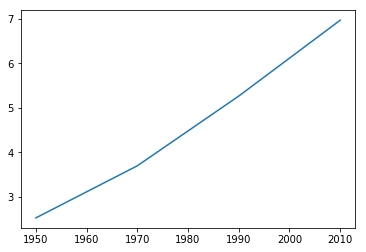## Scatter plot¶

In :
plt.scatter(year, pop)
plt.show()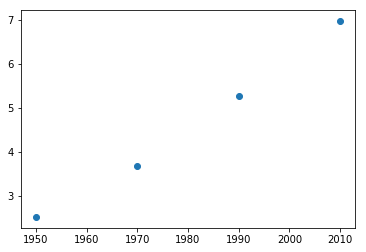# 5.2. Histograms¶

## Histogram¶

• Explore dataset

## Matplotlib¶

In :
import matplotlib.pyplot as plt
help(plt.hist)

Help on function hist in module matplotlib.pyplot:

hist(x, bins=None, range=None, density=None, weights=None, cumulative=False, bottom=None, histtype=u'bar', align=u'mid', orientation=u'vertical', rwidth=None, log=False, color=None, label=None, stacked=False, normed=None, hold=None, data=None, **kwargs)
Plot a histogram.

Compute and draw the histogram of *x*. The return value is a
tuple (*n*, *bins*, *patches*) or ([*n0*, *n1*, ...], *bins*,
[*patches0*, *patches1*,...]) if the input contains multiple
data.

Multiple data can be provided via *x* as a list of datasets
of potentially different length ([*x0*, *x1*, ...]), or as
a 2-D ndarray in which each column is a dataset.  Note that
the ndarray form is transposed relative to the list form.

Masked arrays are not supported at present.

Parameters
----------
x : (n,) array or sequence of (n,) arrays
Input values, this takes either a single array or a sequency of
arrays which are not required to be of the same length

bins : integer or array_like or 'auto', optional
If an integer is given, bins + 1 bin edges are returned,
consistently with :func:numpy.histogram for numpy version >=
1.3.

Unequally spaced bins are supported if *bins* is a sequence.

If Numpy 1.11 is installed, may also be 'auto'.

Default is taken from the rcParam hist.bins.

range : tuple or None, optional
The lower and upper range of the bins. Lower and upper outliers
are ignored. If not provided, *range* is (x.min(), x.max()).
Range has no effect if *bins* is a sequence.

If *bins* is a sequence or *range* is specified, autoscaling
is based on the specified bin range instead of the
range of x.

Default is None

density : boolean, optional
If True, the first element of the return tuple will
be the counts normalized to form a probability density, i.e.,
the area (or integral) under the histogram will sum to 1.
This is achieved by dividing the count by the number of
observations times the bin width and not dividing by the total
number of observations. If *stacked* is also True, the sum of
the histograms is normalized to 1.

Default is None for both *normed* and *density*. If either is
set, then that value will be used. If neither are set, then the
args will be treated as False.

If both *density* and *normed* are set an error is raised.

weights : (n, ) array_like or None, optional
An array of weights, of the same shape as *x*.  Each value in *x*
only contributes its associated weight towards the bin count
(instead of 1).  If *normed* or *density* is True,
the weights are normalized, so that the integral of the density
over the range remains 1.

Default is None

cumulative : boolean, optional
If True, then a histogram is computed where each bin gives the
counts in that bin plus all bins for smaller values. The last bin
gives the total number of datapoints. If *normed* or *density*
is also True then the histogram is normalized such that the
last bin equals 1. If *cumulative* evaluates to less than 0
(e.g., -1), the direction of accumulation is reversed.
In this case, if *normed* and/or *density* is also True, then
the histogram is normalized such that the first bin equals 1.

Default is False

bottom : array_like, scalar, or None
Location of the bottom baseline of each bin.  If a scalar,
the base line for each bin is shifted by the same amount.
If an array, each bin is shifted independently and the length
of bottom must match the number of bins.  If None, defaults to 0.

Default is None

histtype : {'bar', 'barstacked', 'step',  'stepfilled'}, optional
The type of histogram to draw.

- 'bar' is a traditional bar-type histogram.  If multiple data
are given the bars are aranged side by side.

- 'barstacked' is a bar-type histogram where multiple
data are stacked on top of each other.

- 'step' generates a lineplot that is by default
unfilled.

- 'stepfilled' generates a lineplot that is by default
filled.

Default is 'bar'

align : {'left', 'mid', 'right'}, optional
Controls how the histogram is plotted.

- 'left': bars are centered on the left bin edges.

- 'mid': bars are centered between the bin edges.

- 'right': bars are centered on the right bin edges.

Default is 'mid'

orientation : {'horizontal', 'vertical'}, optional
If 'horizontal', ~matplotlib.pyplot.barh will be used for
bar-type histograms and the *bottom* kwarg will be the left edges.

rwidth : scalar or None, optional
The relative width of the bars as a fraction of the bin width.  If
None, automatically compute the width.

Ignored if *histtype* is 'step' or 'stepfilled'.

Default is None

log : boolean, optional
If True, the histogram axis will be set to a log scale. If
*log* is True and *x* is a 1D array, empty bins will be
filtered out and only the non-empty (n, bins, patches)
will be returned.

Default is False

color : color or array_like of colors or None, optional
Color spec or sequence of color specs, one per dataset.  Default
(None) uses the standard line color sequence.

Default is None

label : string or None, optional
String, or sequence of strings to match multiple datasets.  Bar
charts yield multiple patches per dataset, but only the first gets
the label, so that the legend command will work as expected.

default is None

stacked : boolean, optional
If True, multiple data are stacked on top of each other If
False multiple data are aranged side by side if histtype is
'bar' or on top of each other if histtype is 'step'

Default is False

Returns
-------
n : array or list of arrays
The values of the histogram bins. See *normed* or *density*
and *weights* for a description of the possible semantics.
If input *x* is an array, then this is an array of length
*nbins*. If input is a sequence arrays
[data1, data2,..], then this is a list of arrays with
the values of the histograms for each of the arrays in the
same order.

bins : array
The edges of the bins. Length nbins + 1 (nbins left edges and right
edge of last bin).  Always a single array even when multiple data
sets are passed in.

patches : list or list of lists
Silent list of individual patches used to create the histogram
or list of such list if multiple input datasets.

Other Parameters
----------------
**kwargs : ~matplotlib.patches.Patch properties

--------
hist2d : 2D histograms

Notes
-----
Until numpy release 1.5, the underlying numpy histogram function was
incorrect with normed=True if bin sizes were unequal.  MPL
inherited that error. It is now corrected within MPL when using
earlier numpy versions.

.. note::
In addition to the above described arguments, this function can take a
**data** keyword argument. If such a **data** argument is given, the
following arguments are replaced by **data[<arg>]**:

* All arguments with the following names: 'weights', 'x'.



## Matplotlib Example¶

In :
values = [0,0.6,1.4,1.6,2.2,2.5,2.6,3.2,3.5,3.9,4.2,6]
plt.hist(values, bins = 3)
plt.show()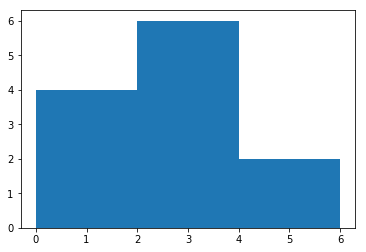# 5.3. Customization¶

## Data Visualization¶

• Science & Art
• Many options
• Different plot types
• Many customizations
• Choice depends on:
• Data
• Story you want to tell

## Basic Plot¶

In :
year = np.linspace(1950.,2100.,num=50)

K = 11.;
P0 = 2.6;
r = 0.03;
population = K*P0*np.exp(r*(year-year)) / (K + P0*(np.exp(r*(year-year))-1.))

plt.plot(year, population)
plt.show()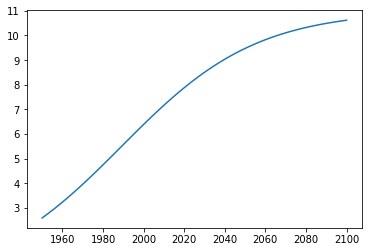## Axis Labels, Title, Ticks, Axis Limits¶

In :
plt.plot(year, population)

plt.xlabel('Year')
plt.ylabel('Population')
plt.title('World Population Projections')
plt.yticks([0,2,4,6,8,10])
plt.xlim(1950, 2100)
plt.ylim(0, 11)

plt.show()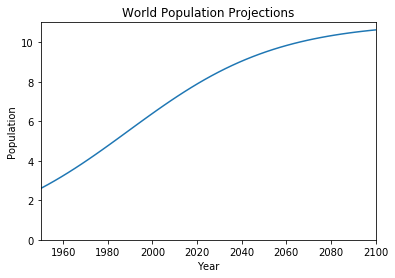## Tick Labels¶

In :
plt.plot(year, population)

plt.xlabel('Year')
plt.ylabel('Population')
plt.title('World Population Projections')
plt.yticks([0,2,4,6,8,10],['0','2B','4B','6B','8B','10B'])
plt.xlim(1950, 2100)
plt.ylim(0, 11)

plt.show()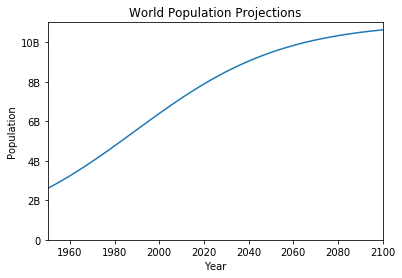# 6.1. Boolean Logic & Control Flow¶

## Booleans¶

In :
2 < 3

Out:
True
In :
2 == 3

Out:
False
In :
x = 2
y = 3
x < y

Out:
True
In :
x == y

Out:
False

## Relational Operators¶

operator meaning
< strictly less than
<= less than or equal
> strictly greater than
>= greater than or equal
== equal
!= not equal

## Logical Operators¶

• and
• or
• not
In :
print(True and True)
print(True and False)
print(False and True)
print(False and False)

True
False
False
False

In :
print(True or True)
print(True or False)
print(False or True)
print(False or False)

True
True
True
False

In :
print(not True)
print(not False)

False
True


## Conditional Statements¶

if condition :
expression


Note the indentation of expression and the colon after the condition.

In :
z = 4
if z % 2 == 0 :
print("z is even")

z is even

In :
z = 5
if z % 2 == 0 :
print("z is even")
else :
print("z is odd")

z is odd

In :
z = 3
if z % 2 == 0 :
print("z is divisible by 2")
elif z % 3 == 0 :
print("z is divisible by 3")
else :
print("z is neither divisible by 2 nor by 3")

z is divisible by 3

In [ ]: